# Parts of Circle

Print
Rate 0 stars
• Lesson size:
Message preview:
Someone you know has shared lesson with you:

To play this lesson, click on the link below:

https://www.turtlediary.com/lesson/parts-of-circle.html

Hope you have a good experience with this site and recommend to your friends too.

Login to rate activities and track progress.
Login to rate activities and track progress.

## Center

In a circle, every point on the circle is at the same distance from the center point.

The center point helps in recognizing the circle.

As you can probably guess from the name, a circle with center O.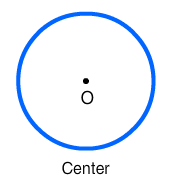The radius of a circle is a line segment that goes from the center point to a point on the circle. It is generally represented as 'r'.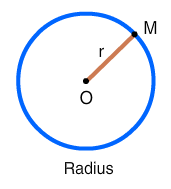A circle has many radii (that's the plural of radius) as you can draw many different lines from the center point to a point on the circle. Each radius is of same length.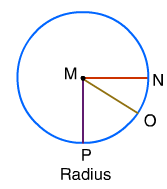In this picture, each radius (MN, MO, MP) has the same length because the distance from the center point to the circle is always the same throughout the circle.

## Diameter

The diameter of circle is a line segment that goes all the way across a circle through the center point. It is the longest distance across the circle as it passes through the centre. It is represented as 'd'.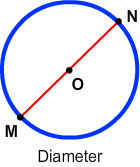A circle actually has many diameters since you can draw many different lines through the center of the circle. Each diameter, however, has the same length.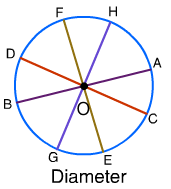In this picture, each diameter (MN, MO, MP) has the same length because all diameters of a circle have the same length, this being twice the radius.

So, diameter = 2 x radius

or               d = 2 x r

## Circumference

The circumference of a circle is the distance around the outer edge of the circle. It is really a fancy name for the perimeter of the circle.

Circumference = 2 x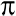x r where= 3.14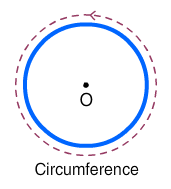If you were to run around a circular track, the distance you ran would be the circumference of the circular track.

## Chord

A chord is any line segment that connects any two points on the circle.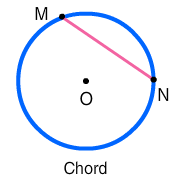Since the diameter connects two points on the circle, it is also a chord. It is the largest chord in the circle because it goes all the way across through the center.

Every diameter is chord but every chord is not a diameter.

## Arc

An arc is any connected part of the circle.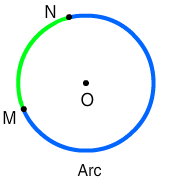An arc is a segment or a part of the circumference of the circle. It is always curved since circles are curved.

### Example 1

Identify the different parts of the circle.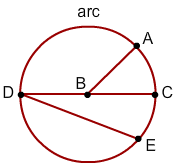In this picture,

• Point B is the center point of the circle.
• AB is a radius because it start from the center B to a point A on the circle.
• DC is a diameter because it goes all the way across the circle through the center B.
• DC and DE are the chords since it connects two points on the circle. DE is NOT a diameter because it does not go through the center.
• The distance all the way around the circle is always the circumference.
• AC is an arc because it is a connected part of the circle.

## Parts of Circle

Various parts of a circle

1. Center point
It is the center of the circle. Every point on the circle is at the same distance from the center.2. Diameter
A line segment that goes all the way across a circle through the center point.A line segment that goes from the center point to a point on the circle. Thus, it goes halfway through the circle.4. Circumference
The distance around the outer edge of the circle.5. Chord
A line segment that connects any two points on the circle.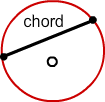6. Arc
Any connected part of the circle.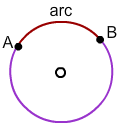## Similar Lessons

Become premium member to get unlimited access.